Algebra 2 7-2 Guided Practice: Properties of Exponential Functions
starstarstarstarstarstarstarstarstarstar
by Matthew Richardson
| 9 Questions1
10
Solve It! f and g are exponential functions with the same base.

Is the graph of g
● a compression,
● a reflection, or
● a translation
of the graph of f ? Or is it none of the above? Select all that apply.
A compression
A reflection
A translation
None of the above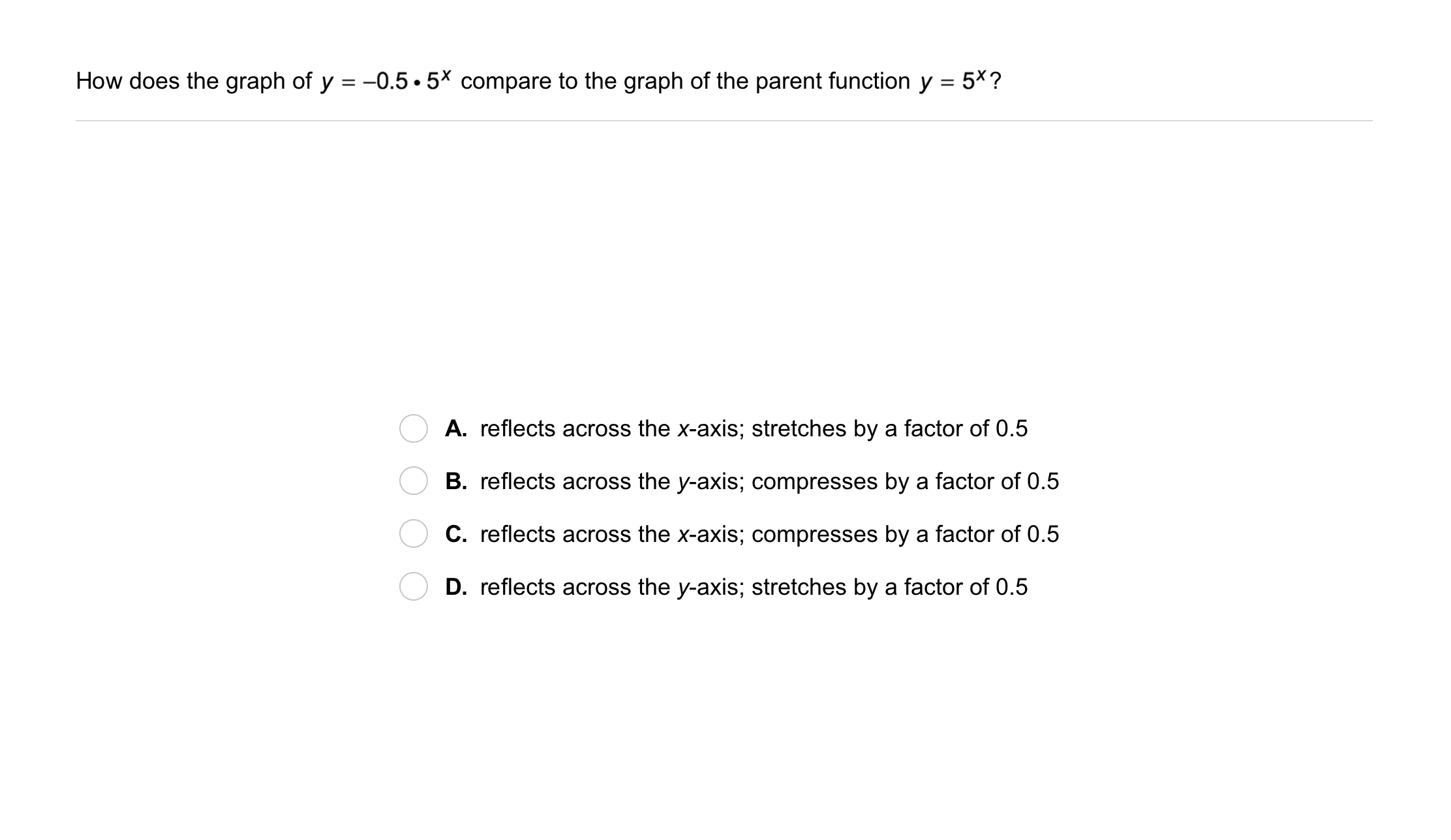2
2
10
Problem 1 Got It?
A
B
C
D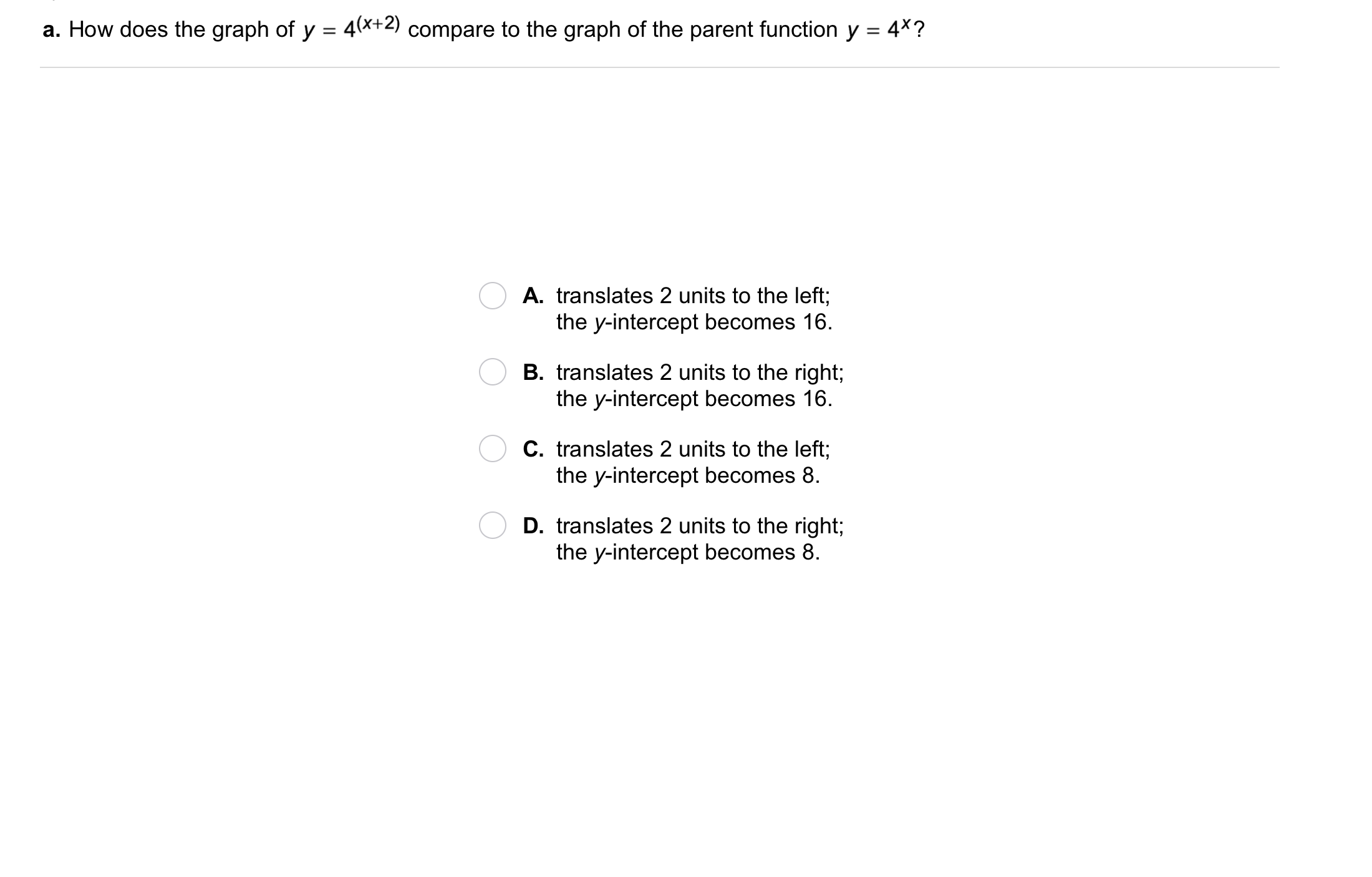3
3
10
Problem 2 Got It?
A
B
C
D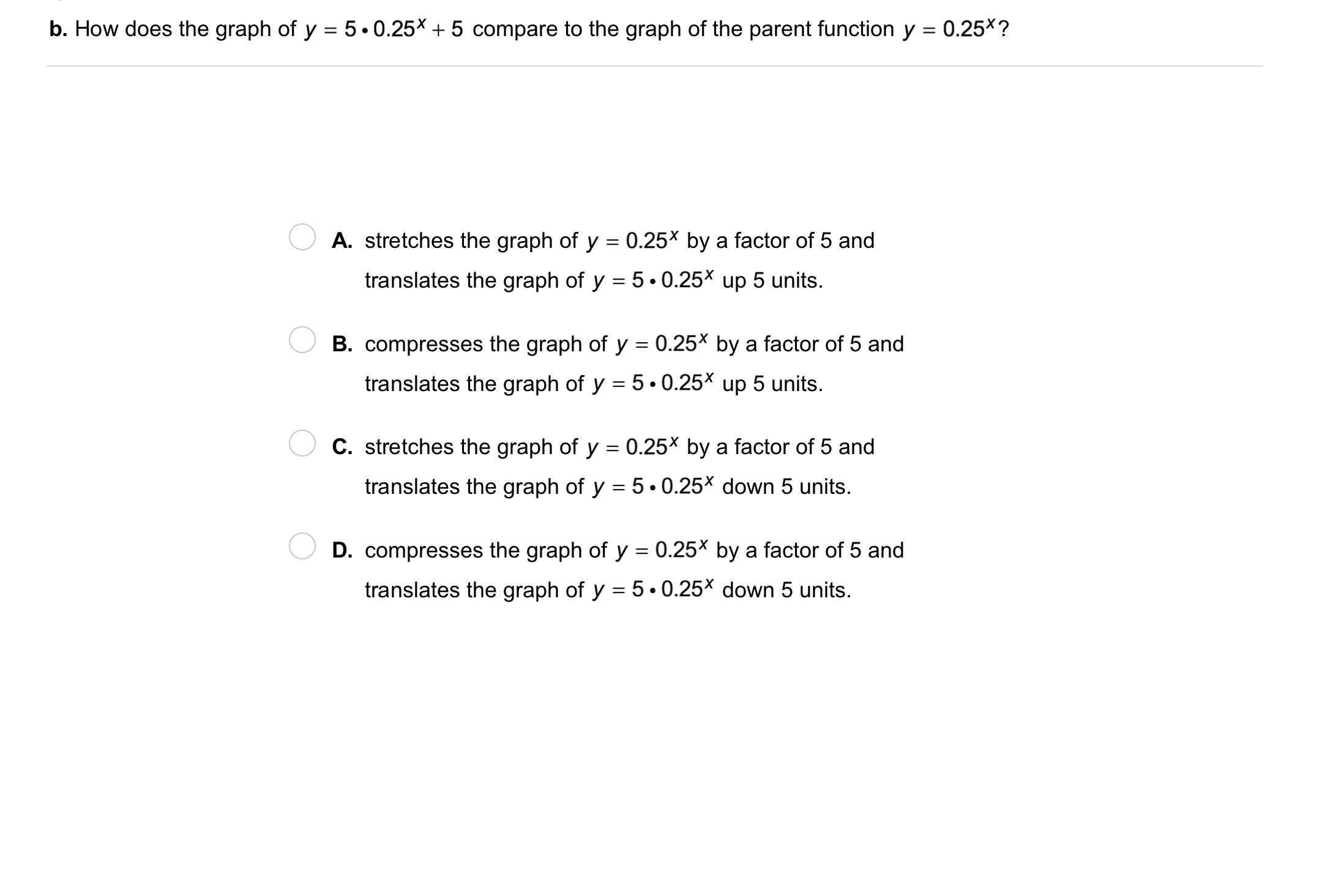4
4
10
Problem 2 Got It?
A
B
C
D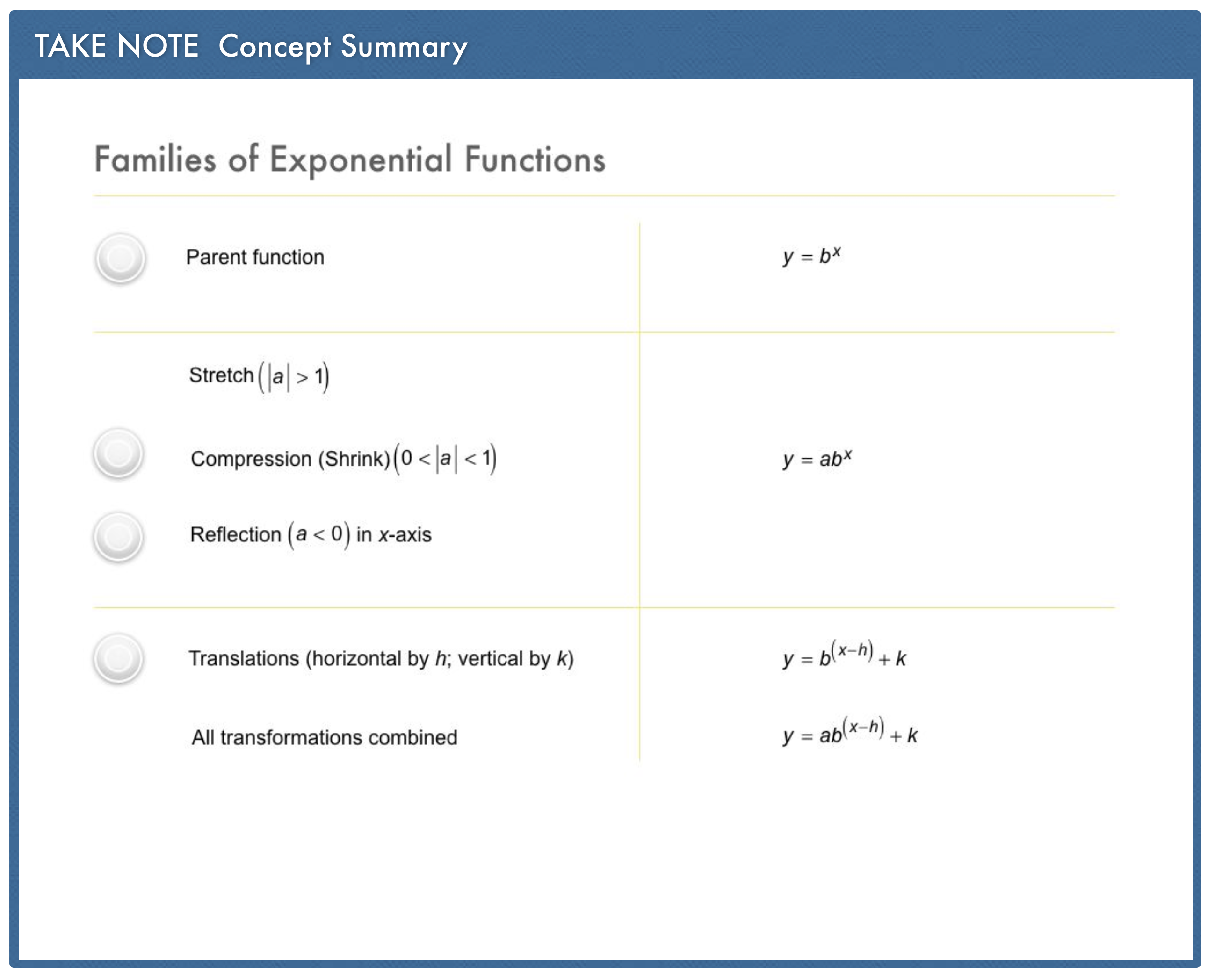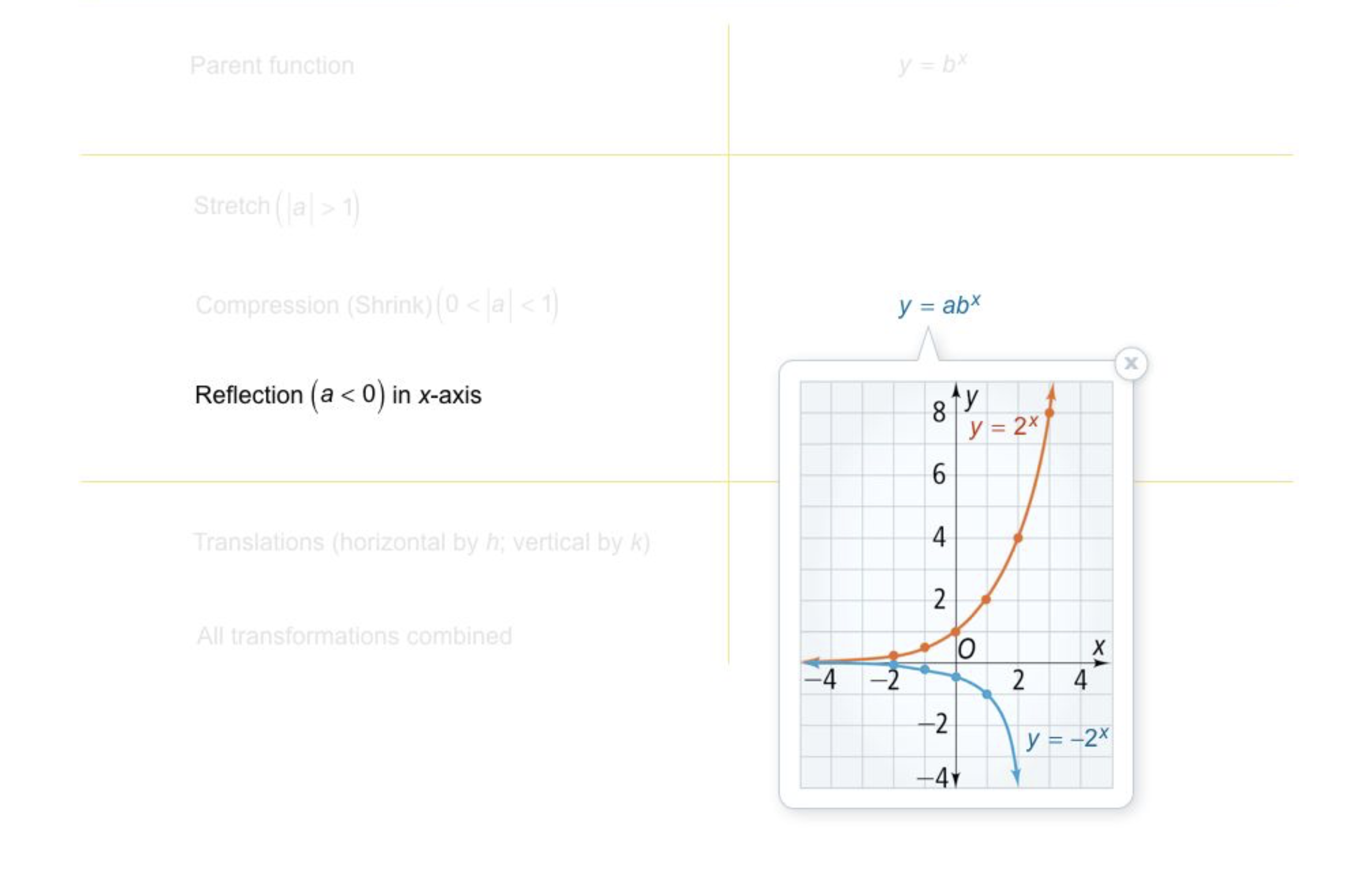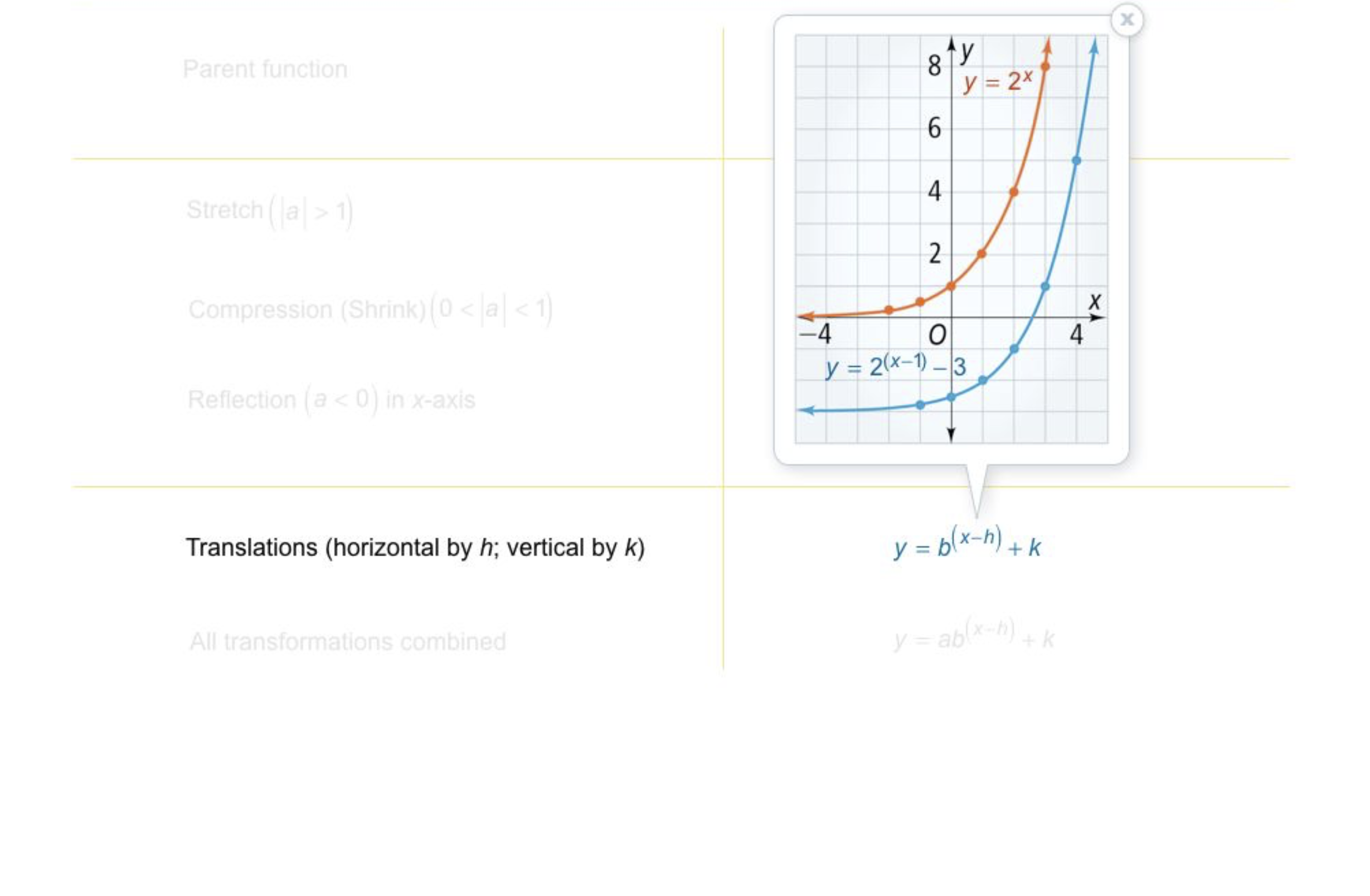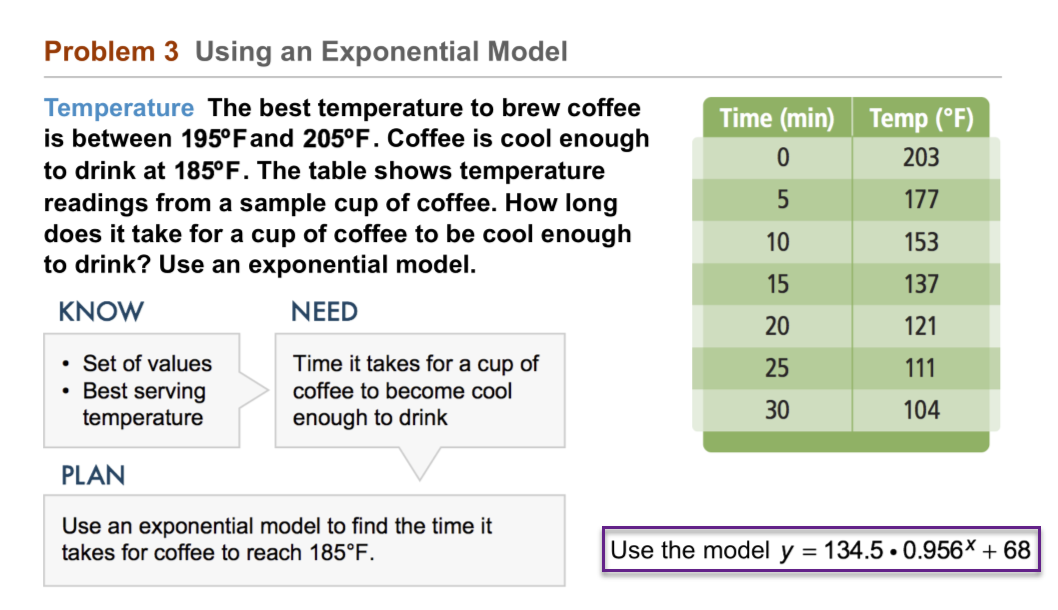5
10
Problem 3 Got It? Use the exponential model from Problem 3.
How long does it take for the coffee to reach a temperature of 100 degrees?
6
10
Problem 3 Got It? Reasoning: In Problem 3, would the model of the exponential data be useful if you did not translate the data by 68 units? Explain.
Yes; since a hot drink in this scenario may cool well below room temperature, it is not necessary to translate the data.
No; a hot drink in this scenario will not cool below room temperature. In order to use exponential data, it is important to translate the data left 68 units.
No; a hot drink in this scenario will not cool below room temperature. In order to use exponential data, it is important to translate the data up 68 units.7
10
Problem 4 Got It? How can you use a graphing calculator to calculate e8?
Use the ex key, x = 8
Graph y = ex and find y for x = 8
Use the table of values for y = ex and find y for x = 8
All of the above
8
10
Problem 4 Got It? Calculate e8. Round to 5 decimal places. Enter only a number with no commas or spaces.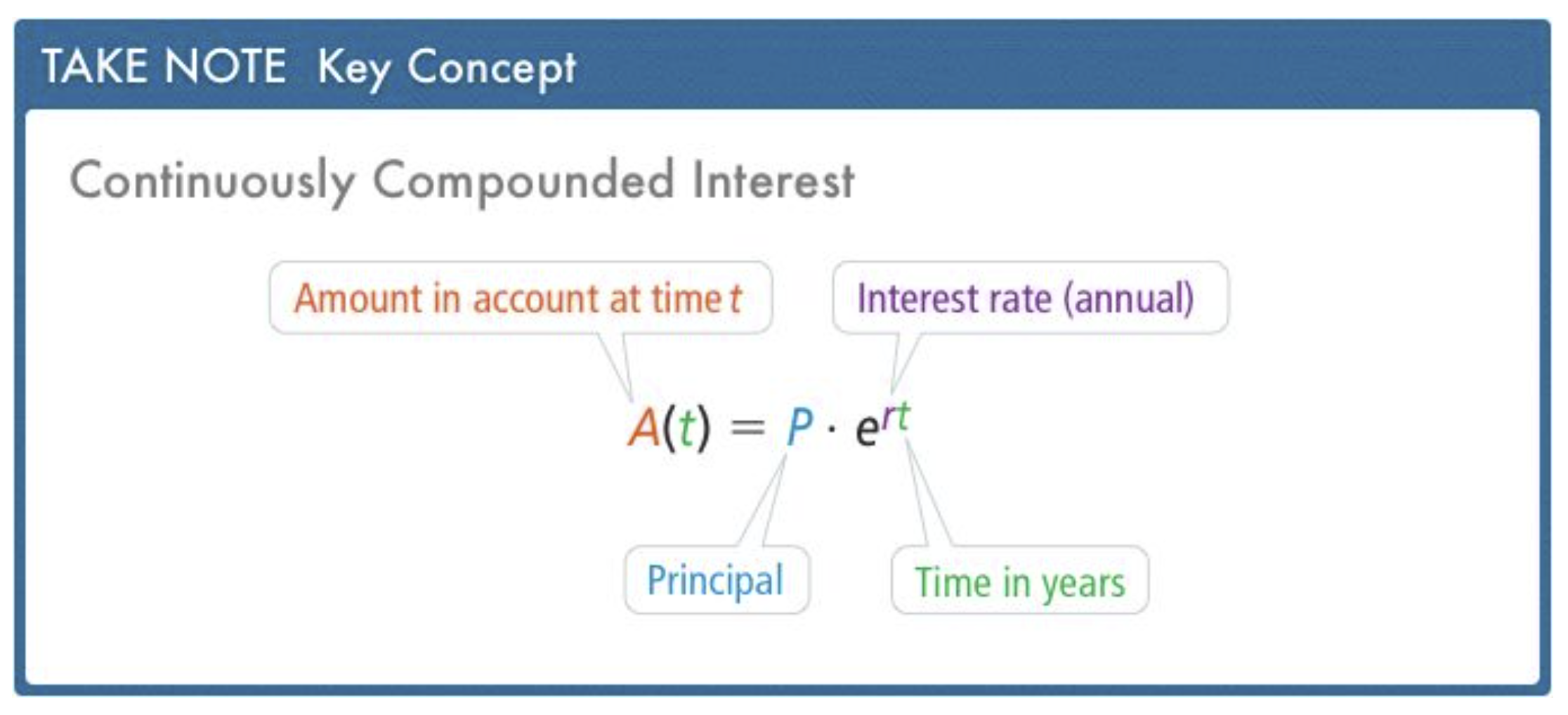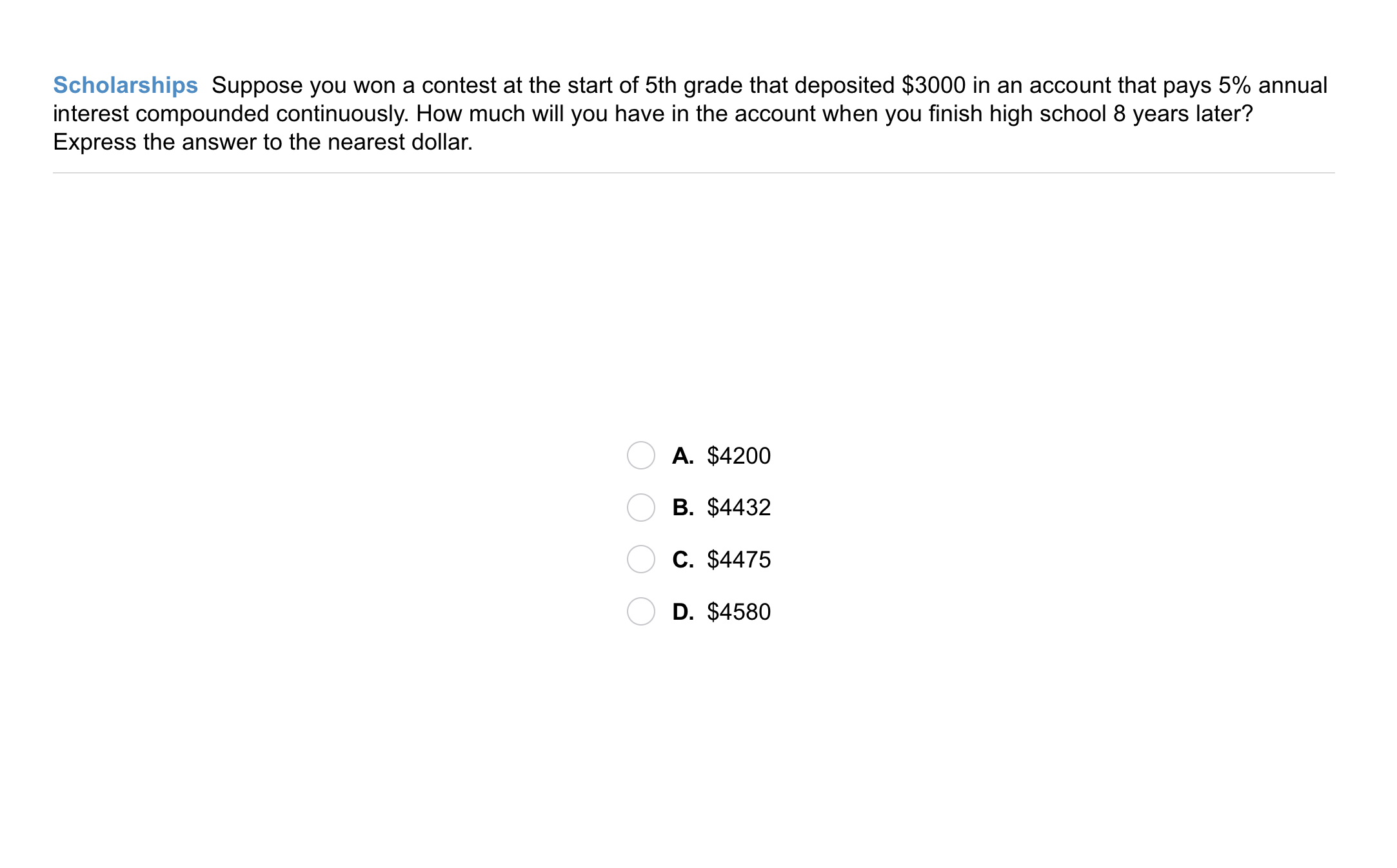9
9
10
Problem 5 Got It? Scholarships: Suppose you won a contest at the start of 5th grade that deposited \$3000 in an account that pays 5% annual interest compounded continuously. How much will you have in the account when you finish high school 8 years later? Express the answer to the nearest dollar.
A
B
C
D